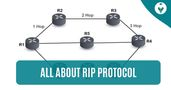Function in C | About, Types and Examples

# Function in C | About, Types and Examples

clickHere
Updated on Nov 28, 2023 11:02 IST

Have you heard of functions in the C programming language? Let's explore it, including its types and examples in detail!

A function in C is a collection of statements designed to execute a specific task when invoked. These self-contained units of code, often referred to as subroutines or procedures in other programming languages, play a pivotal role in structuring a C program. They provide a way to break down a program into smaller, manageable units, which makes the code more readable, reusable, and maintainable.

Also, explore Function Prototype in C

Let’s see the types of Functions below:

## Syntax of Function in C

```return_type function_name(parameter_list) {
// body of the function
// ...
return return_value;
}```

### Let us understand each part of the syntax in detail

return_type: This specifies the type of data that the function will return. It can be any valid data type in C such as int, float, char, etc., or void if the function does not return any value.

function_name: This is the name of the function, which you use to call the function elsewhere in your program. It can be any valid identifier name in C.

parameter_list: This is a list of parameters that the function takes as input. Parameters are specified as a comma-separated list of type name pairs, such as int a, float b. If the function takes no parameters, you can use the keyword void or leave it empty.

The body of the function: This is a block of code enclosed in curly braces {}. This is where you write the code that implements the functionality of the function.

return: This keyword is used to return a value from the function. The type of the value being returned must match the return_type specified in the function definition. If the return_type is void, you can simply use the return; statement without any value, or omit the return statement entirely.

### Exampleto understand the above part

```#include <stdio.h> // Function definitionint add_numbers(int a, int b) {  // int is the return type, add_numbers is the function name, and it takes two parameters of type int    int sum;                     // local variable to hold the sum of the two numbers    sum = a + b;                 // calculating the sum of the two numbers    return sum;                  // returning the sum} int main() {    int result = add_numbers(7, 3);  // Calling the function with 5 and 3 as arguments    printf("The result is: %d\n", result);  // Printing the result    return 0;  // Returning 0 to indicate successful execution}Copy code```

### Output

`The result is: 10`

## Let’s Understand Functions by Relating themWith a Real-Life Example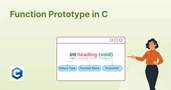Function Prototype in C
This article defines the scope of function prototype Importance, examples of Function Prototype and Difference between Function Prototype and Function Definition in C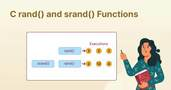What are C rand() and srand() Functions?
The purpose of this blog is to make you understand the rand() and sand() C programming functions. Let’s learn about them with the help of easy examples.User Defined Functions in C | Meaning, Types and Examples
In this blog, we will discuss about User Defined Functions in C in detail from scratch! First we will understand what are functions & then, jump into the world of...read more

Imagine a software product that manages a library’s book inventory. Here’s a small example demonstrating a function

```#include <stdio.h> typedef struct {    char title;    char author;    int year_published;} Book; void display_book_details(Book book) {    printf("Title: %s\n", book.title);    printf("Author: %s\n", book.author);    printf("Year Published: %d\n", book.year_published);} int main() {    Book myBook = {"One Hundred Years of Solitude", "Gabriel García Márquez", 1967};     display_book_details(myBook);     return 0;}Copy code```

Output

```Title: One Hundred Years of Solitude
Author: Gabriel García Márquez
Year Published: 1967```

In the above example:

1. Function Definition: display_book_details is a function defined to display the details of a book.
2. Return Type: The function has a void return type because it does not return any value; it only prints details.
3. Function Name: display_book_details is the name of the function.
4. Parameters: The function takes a Book structure as a parameter, which contains details about a book.
5. Calling the Function: The function is called from the main function with myBook as the argument.

So, in this product, the display_book_details function can be seen as a tool that library staff use to quickly display details of a book in the inventory, helping them to manage and catalog books more efficiently.

## Let’s Understand the Types of Functions in Detail:

### 1. Built-in Functions

Built-in functions in C, often referred to as library functions or standard library functions, are predefined functions that are part of the C standard library. These functions provide essential capabilities to C programmers and can be used without the need for writing their implementations.

Some types of Built-in Functions with examples

1. String Manipulation Functions (<string.h>)

These functions provide various ways to manipulate and work with strings.

• strcpy(): Copies one string into another.
• strlen(): Returns the length of a string.
• strcmp(): Compares two strings.
• strcat(): Concatenates two strings.

Example

```#include <stdio.h>#include <string.h> int main() {    char source[] = "Hello, Learn C Programming with Shiksha Online!";    char destination;     strcpy(destination, source);     printf("Source String: %s\n", source);    printf("Destination String: %s\n", destination);     return 0;}Copy code```

Output

```Source String: Hello, Learn C Programming with Shiksha Online!
Destination String: Hello, Learn C Programming with Shiksha Online!```

In this example, strcpy() is used to copy the content of source string into destination string. It illustrates the importance of being able to copy strings safely and easily in C programming.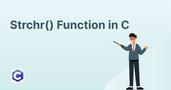Understanding Strchr() Function in C
This blog explains the strchr() Function in C programming with the help of examples. Let’s understand!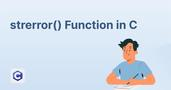Understanding Strerror() Function in C
This blog covers strerror() Function in C programming. We have explained this concept with the help of examples.Explore toupper() Function in C with Examples
The toupper() function takes a single character as input and returns the corresponding uppercase character. It is commonly used in conjunction with string manipulation functions to convert entire strings to...read more

2. Mathematical Functions (<math.h>)

• pow(): Returns the power of a number.
• sqrt(): Returns the square root of a number.
• sin(), cos(), tan(): Trigonometric functions.
• log(): Returns the natural logarithm of a number.

Example

```#include <stdio.h>#include <math.h> int main() {    double number = 81.0;    double result = sqrt(number);     printf("The square root of %.2f is %.2f\n", number, result);     return 0;}Copy code```

Output

```The square root of 81.00 is 9.00
```

Here, sqrt() is used to find the square root of a number. This kind of function is vital in scientific computing, game development, and other fields where mathematical operations are commonly used.

3. Input and Output Functions(<stdio.h>)

Functions for input and output operations, mainly dealing with file and console I/O.

• printf(): Outputs formatted string to the console.
• scanf(): Reads input from the console.
• fopen

clickHere

I'm Esha Gupta, a B.Tech graduate in Computer Science & Engineering, specializing in Front End Web Dev. I've interned at GeeksforGeeks & Coding Minutes, fueling my passion for crafting appealing and functional digit... Read Full Bio

## Trending Technology CoursesC Programming For Beginners - Master the C LanguageC Programming For Beginners - Master the C Languag...
UDEMY4.6C for Everyone: Programming Fundamentals
Coursera4.6C for Everyone: Programming Fundamentals
Coursera4.6Starts today## Top Picks & New Arrivals